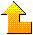The 19th Finnish Summer School on Probability Theory

# The 19th Finnish Summer School on Probability Theory

19. todennäköisyyslaskennan kesäkoulu pidetään Lahden Mukkulassa 2-6.6.1997 (tiedotus ja ilmoittautumislomake suomeksi)

19 sommarskolan i sannolikhetsteori hålls på Mokulla gård i Lahtis den 2-6 juni 1997 (information och anmälningsblankett pĺ svenska)

The 19th Finnish Summer School on Probability Theory will be arranged at Lahti from June 2 to June 6, 1997. The main speakers:

More information and lecture abstracts will be distributed in April - May. There is no participation fee, but we need to limit the number of participants to about 30.

## Course description

Jean Bertoin sent us a tentative programme:

### Tentative Programme

#### I. Lévy processes and the Lévy-Khintchine formula

• 1. Preliminaries on Poisson point processes (definition of PPP, counting processes, martingales in PPP, exponential formula)
• 2. The Lévy-Khintchine formula and the Lévy-Itô decomposition
• (definition of Lévy processes, infinitely divisible laws, Lévy-Khintchine formula, Lévy-Itô decomposition of a Lévy process)
• 3. Lévy processes as Markov processes (Markov property, semigroup, Feller property, resolvent, infinitesimal generator, formulas with Fourier transform, transience and recurrence)
• #### II. Subordinators

• 1. Definition and basic properties (Examples, Laplace exponent, renewal function, estimates)
• 2. First passage times (distribution of a first passage time, regularly varying functions and the Dynkin-Lamperti theorem)
• 3. Fractal properties of the range (the range of a subordinator, various notions of fractal dimensions, Hausdorff and packing dimensions)
• 4. Regenerative sets (regenerative property of the range, local time of a regenerative set, case of Markov processes)
• #### III. Fluctuation theory

• 1. Reflected Lévy process (extrema, Markov property of the reflected process, ladder process and subordinators)
• 2. Fluctuation identities (Spitzer-Rogozin identity, Fristedt formula, Baxter-Donsker formula, case of stable processes)
• 3. Spectrally negative Lévy processes (fluctuation identities, the two-sided exit problem)
• #### IV. On the Arcsine laws

• 1. Spitzer's theorem (occupation time of $[0,\infty)$, Sparre-Andersen identity, Spitzer's condition, the arcsine theorem, estimate for the first passage time)
• 2. Doney's condition (expressions for $P(X_t\geq 0)$, equivalence of Spitzer and Doney's condition)
• 3. Sample path behaviour of the last ladder epoch (comparison of size of a subordinator and its jumps, a.s. behaviour related to the Arcsine theorem)
• 4. Sample path behaviour of the occupation time of $[0,\infty)$ (another a.s. behaviour related to the Arcsine theorem)
• Tomas Björk:

### Mathematical Finance

#### Lecture plan:

• 1. Introduction to financial derivatives. The binomial model.
• 2. Financial markets in continuous time. Absence of arbitrage and martingale measures.
• Completeness and stochastic integral representations. The Black-Scholes model.
• 3. Introductory interest rate theory. The term structure equation.
• Affine Term Structures. Calibration of models to market data.
• 4. Forward rate modelling. The Heath-Jarrow-Morton approach.
• 5. Change of numeraire.
• 6. New directions.
• Literature:
Bjork, T: Interest Rate Theory. (In W. Runggaldier (ed): "Financial Mathematics" Springer Lecture Notes Vol. 1656.)

Copies of the lecture overheads will be handed out.

Dave Donoho describes his lectures as follows:

Wavelets and Beyond (with applications in Statistics and Probability)

The last ten years have seen an explosive development of new representations and expansions: new bases, frames, and atomic decompositions, of which wavelets are only the most well known. In this series of lectures we will give a basic introduction to wavelets, their mathematical and computational properties, applications in statistics, and an introduction to software packages for using them. We will try to describe the needs in harmonic analysis to which they respond and the connection with modern technological developments (data compression).

Then we will discuss why wavelets are only 'the tip of the iceberg' and discuss the myriad of new developments which wavelets have spawned, which offer new insights and challenges.

Our lectures will cover the following topics

• Wavelets-Basic Introduction
• Wavelets and Computation
• Wavelets and Statistics
• Nonparametric Estimation
• Nonparametric Inference
• Wavelets and Probability
• Representation of Stochastic Processes
• What's so Special about Wavelets?
• The problem of optimal representation

Beyond Wavelets-Modern Computational Harmonic Analysis Time Frequency Analysis-Wavelet Packets, Cosine Packets, Chirplets Image Analysis-Steerable Pyramids, Brushlets, Edgelets, Wedgelets What are the real achievements? Challenges?

Saul Jacka writes the following:

#### Applications of Stochastic Control and Optimal Stopping

The talks will concentrate on the application of the techniques of stochastic control in Probability and Finance.

The following is a very tentative schedule for the talks.

• 1. Introduction: Sub/super martingale arguments and the HBJ equation. Krylov's conditions. Optimal stopping and the Snell envelope; free-boundary problems and smooth-pasting. Control via the Girsanov transform.
• 2. Stochastic control in finance: optimal portfolio selection and consumption problems.
• 3. Tracking problems: tracking a diffusion by controlling the diffusion parameter and finite-fuel control problems.
• 4. Optimal stopping: application to pricing American options and to the good-lambda inequalities.
• 5. Further applications of the Lagrangian/penalisation method: best constants in inequalities via optimal stopping.
• #### Time Permitting:

• 6. EITHER control of measure-valued diffusions with applications to the compact support property OR properties of optimal stopping regions and smooth-pasting results via local-time.

#### Train Helsinki-Kouvola-KotkaTo The Department of Mathematics' Homepage
Updated 14.5.97 by Ann-Mari.Dahlstrom@abo.fi